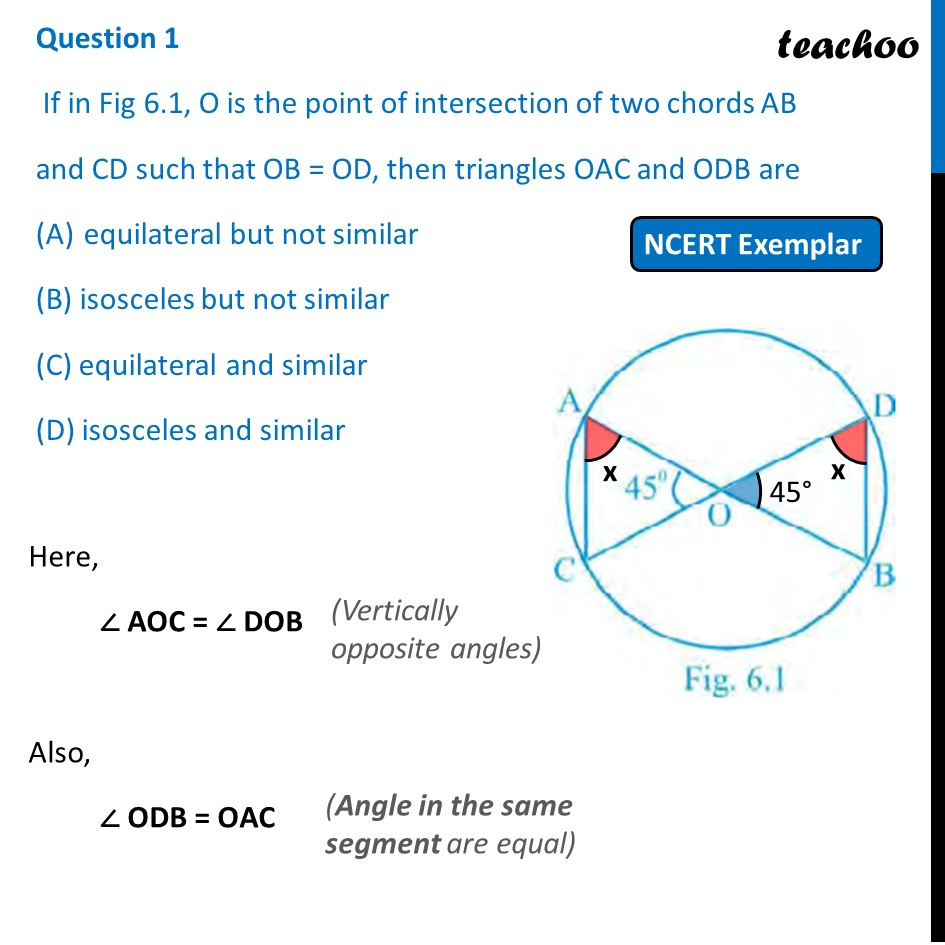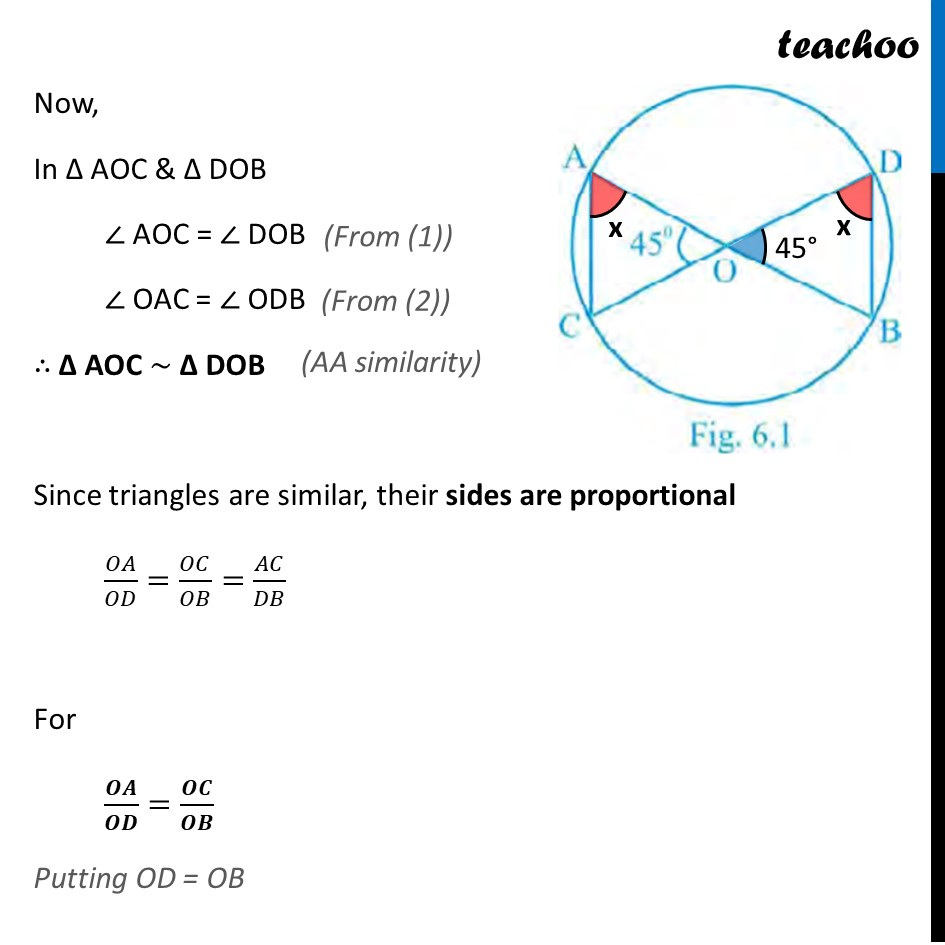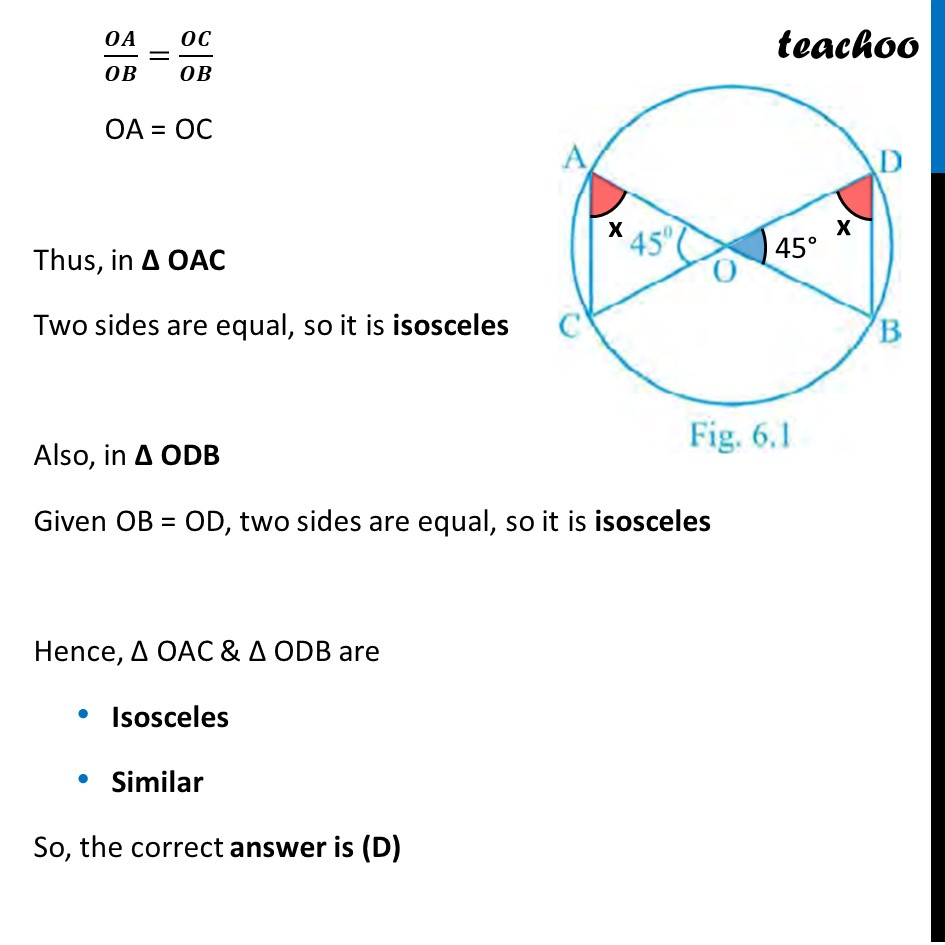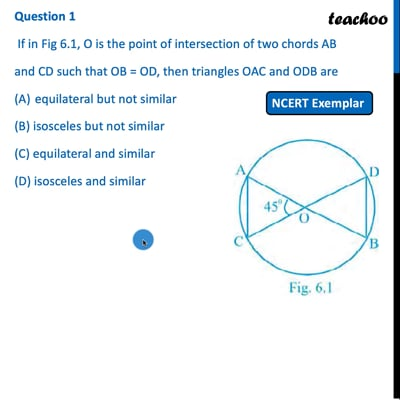NCERT Exemplar - MCQ

Chapter 6 Class 10 Triangles
Serial order wise

## (D) isosceles and similarThis video is only available for Teachoo black users

Introducing your new favourite teacher - Teachoo Black, at only ₹83 per month

### Transcript

Question 1 If in Fig 6.1, O is the point of intersection of two chords AB and CD such that OB = OD, then triangles OAC and ODB are equilateral but not similar (B) isosceles but not similar (C) equilateral and similar (D) isosceles and similar Here, ∠ AOC = ∠ DOB Also, ∠ ODB = OAC (Vertically opposite angles) (Angle in the same segment are equal) Now, In Δ AOC & Δ DOB ∠ AOC = ∠ DOB ∠ OAC = ∠ ODB ∴ Δ AOC ~ Δ DOB Since triangles are similar, their sides are proportional 𝑂𝐴/𝑂𝐷=𝑂𝐶/𝑂𝐵=𝐴𝐶/𝐷𝐵 For 𝑶𝑨/𝑶𝑫=𝑶𝑪/𝑶𝑩 Putting OD = OB (From (1)) (From (2)) (AA similarity) 𝑶𝑨/𝑶𝑩=𝑶𝑪/𝑶𝑩 OA = OC Thus, in Δ OAC Two sides are equal, so it is isosceles Also, in Δ ODB Given OB = OD, two sides are equal, so it is isosceles Hence, Δ OAC & Δ ODB are Isosceles Similar So, the correct answer is (D)